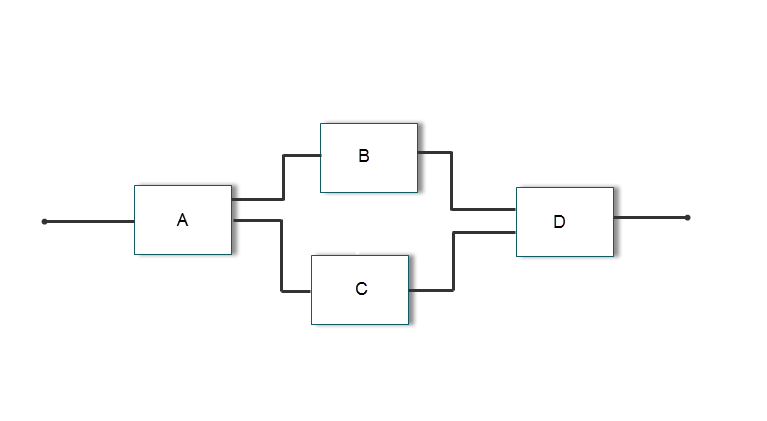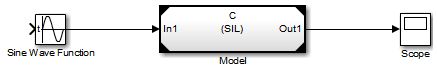## Choose a SIL or PIL Approach

Consider a top model that consists of components `A`, `B`, `C`, and `D`:

• `A` and `B` are existing components for which code has previously been generated and tested.

• `C`, a referenced model, and `D`, a subsystem, are new components.With software-in-the-loop (SIL) and processor-in-the-loop (PIL) simulations, you can use the following approaches to numerical equivalence testing:

For some forms of testing, you require a test harness model. The test harness model:

• Generates test vectors or stimulus inputs that feed the block under test.

• Makes it possible for you to observe or capture output from the block.

The following example shows a simple test harness model.The block under test is a Model block. The Sine Wave block generates the input for the Model block. Through the Scope block, you can observe the output from the Model block. For information about creating and using test harnesses, see Create Test Harnesses and Select Properties (Simulink Test) and Code Generation Verification Workflow with Simulink Test (Simulink Test).

### Test Top-Model Code

To test code generated from the top-model components together (`A`, `B`, `C`, and `D`), you can use top-model SIL/PIL or Model block SIL/PIL.

• Top-model SIL/PIL:

1. Create test vectors or stimulus inputs in the MATLAB workspace.

2. Run the top model in normal, SIL, and PIL simulation modes. The software loads the test vectors or stimulus inputs from the MATLAB® workspace.

3. For each simulation mode, observe or capture outputs.

4. Verify numerical equivalence by comparing normal outputs against SIL and PIL outputs.

• Model block SIL/PIL:

1. Create a Model block that contains the top-model components.

2. Insert the Model block in a simulation model, for example, your test harness model.

3. Run simulations, switching the Model block between normal, SIL, and PIL modes. For the SIL and PIL simulation modes, set the Code interface Model block parameter to ```Top model```.

4. Verify numerical equivalence by comparing normal outputs against SIL and PIL outputs.

For more information, see Simulation with Top Model and SIL/PIL Manager Verification Workflow.

### Test Referenced Model Code

To test code generated from the component `C` as part of a model reference hierarchy, use the Model block SIL/PIL approach:

• Insert the Model block `C` in a simulation model, for example, your test harness model.

• Run simulations, switching the Model block between normal, SIL, and PIL modes. For the SIL and PIL simulation modes, set the Code interface Model block parameter to ```Model reference```.

• Verify numerical equivalence by comparing normal outputs against SIL and PIL outputs.

For more information, see Simulation with Model Blocks and SIL/PIL Manager Verification Workflow.

### Test Subsystem Code

To test code that is generated from the subsystem `D`, use one of these workflows.

WorkflowDescription
Simulink Test harness with SIL/PIL Manager

Perform unit tests on subsystem code that is part of the code generated from the parent model.

1. Generate code for the model that contains the subsystem by running `slbuild(model)` or ```slbuild(model, 'RTWModelReferenceTargetOnly')```.

2. In the model, right-click the subsystem and then create a test harness for the subsystem.

3. Open the SIL/PIL Manager. If required, enable code coverage analysis. Then run back-to-back model and SIL or PIL simulations.

4. If required, export an equivalence test case.

If the subsystem is not supported by this workflow, use the SIL or PIL block workflow. For more information about unsupported subsystems and other limitations, see Atomic Subsystem Workflow Limitations.

SIL or PIL block

Generate and test new standalone code from the subsystem.

1. Insert the subsystem in a simulation model, for example, your test harness model.

2. Run a normal mode simulation, capturing the outputs.

3. Create a SIL or PIL block from the subsystem.

4. In the model, replace the subsystem with the SIL or PIL block.

5. Run a simulation of the model, capturing the outputs.

6. Verify numerical equivalence by comparing normal mode subsystem outputs against SIL or PIL block outputs.

### Summary

Component From Which Code Is Generated Simulation UsesStepsGenerated Code InterfaceTest Signal Source
Top modelTop-model SIL/PIL

In SIL/PIL Manager:

1. Select SIL/PIL Simulation Only mode.

2. In System Under Test, select `Top model`.

StandaloneMATLAB workspace
Model referenced by Model blockModel block SIL/PIL

1. In SIL/PIL Manager:

1. Select SIL/PIL Simulation Only mode.

2. In System Under Test, select ```Model blocks in SIL/PIL mode```.

2. In Model blocks, set Simulation mode to ```Software-in-the-loop (SIL)``` or ```Processor-in-the-loop (PIL)```.

Determined by Model block parameter Code interface –– standalone or model reference.Simulation model, for example, test harness model
SubsystemSimulink Test harness and SIL/PIL Manager

1. Generate code for model that contains the atomic subsystem.

2. Create a test harness for the subsystem.

3. From SIL/PIL Manager, run back-to-back model and SIL or PIL simulations.

4. If required, export equivalence test case.

Determined by generated parent model code –– standalone or model reference. Simulink® Test™ harness
SubsystemSIL or PIL blockManual block substitutionStandaloneSimulation model, for example, test harness model.